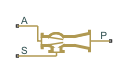# Jet Pump

Jet liquid-liquid pump

• Library:
• Simscape / Fluids / Hydraulics (Isothermal) / Pumps and Motors

•## Description

The Jet Pump block represents a jet liquid-liquid pump consisting of a nozzle, throat, and diffuser, as shown in this illustration.The model is based on these equations, described in :

 ${q}_{1}=\frac{{A}_{n}}{\sqrt{1+{K}_{n}}}\sqrt{\frac{2}{\rho }\left({p}_{1}-{p}_{0}\right)}$ (1)
 ${q}_{2}=\frac{{A}_{n}\cdot c}{\sqrt{1+{K}_{en}}}\sqrt{\frac{2}{\rho }\left({p}_{2}-{p}_{0}\right)}$ (2)
 ${p}_{d}-{p}_{0}=Z{b}^{2}\left(\frac{2}{b}+\frac{2}{1-b}{M}^{2}-{\left(1+M\right)}^{2}\cdot \left(1+{K}_{th}+{K}_{di}+{a}^{2}\right)\right)$ (3)
`$b=\frac{{A}_{n}}{{A}_{th}}$`
`$c=\frac{1-b}{b}$`
`$Z=\rho \frac{{V}_{n}^{2}}{2}=\rho \frac{{q}_{1}^{2}}{2{A}_{n}^{2}}$`
`$M=\frac{{q}_{2}}{{q}_{1}}$`

where:

 q1 Primary flow rate pumped through the nozzle q2 Secondary flow rate qd Output flow rate p1 Pressure at the nozzle inlet p2 Pressure at the secondary flow rate inlet p0 Pressure at the throat inlet pd Pressure at the pump outlet An Nozzle area Ath Throat area a Diffuser area ratio, Ath / Ad Ad Diffuser outlet area Kn Nozzle hydraulic loss coefficient Ken Throat entry hydraulic loss coefficient Kth Throat hydraulic loss coefficient Kdi Diffuser hydraulic loss coefficient ρ Fluid density

Equation 1 describes the nozzle, Equation 2 describes the throat entry, and Equation 3 describes the combination of the throat and the diffuser. The equations correspond to a standard configuration of the pump, where all the longitudinal dimensions conform to established, empirically determined values. For more details, see .

The pump parameters are closely related to each other, and the methodology described in  is recommended to determine their initial values.

### Variables

To set the priority and initial target values for the block variables prior to simulation, use the Initial Targets section in the block dialog box or Property Inspector. For more information, see Set Priority and Initial Target for Block Variables.

Nominal values provide a way to specify the expected magnitude of a variable in a model. Using system scaling based on nominal values increases the simulation robustness. Nominal values can come from different sources, one of which is the Nominal Values section in the block dialog box or Property Inspector. For more information, see Modify Nominal Values for a Block Variable.

## Assumptions and Limitations

• The model is based on the one-dimensional theory.

• The primary and secondary flows enter the mixing throat with uniform velocity distribution, and the mixed flow leaves the diffuser with uniform velocity distribution.

• The fluid in the primary and secondary flows is the same.

• The fluid is assumed to be incompressible and containing no gas.

## Ports

### Conserving

expand all

Hydraulic conserving port associated with the nozzle entry. The nozzle is the primary flow entry.

Hydraulic conserving port associated with the pump suction. The pump suction is the secondary flow entry.

Hydraulic conserving port associated with the pump outlet.

Internal hydraulic conserving port associated with the throat entry section of the pump. This port is not visible on the block icon. You can view the variables associated with the port by logging the simulation data. For more information, see Data Logging.

## Parameters

expand all

Cross-sectional area of the nozzle.

Cross-sectional area of the throat. The throat area is usually two to four times larger than the nozzle area.

Ratio between the inlet and outlet diffuser areas. For a standard pump with a 5°–7° included-angle diffuser, the ratio is close to 0.2.

Hydraulic friction loss coefficient in the nozzle.

Hydraulic friction loss coefficient in the throat entry.

Hydraulic friction loss coefficient in the throat.

Hydraulic friction loss coefficient in the diffuser.

 I.J. Karassic, J.P. Messina, P. Cooper, C.C. Heald, Pump Handbook, Fourth edition, McGraw-Hill, NY, 2008